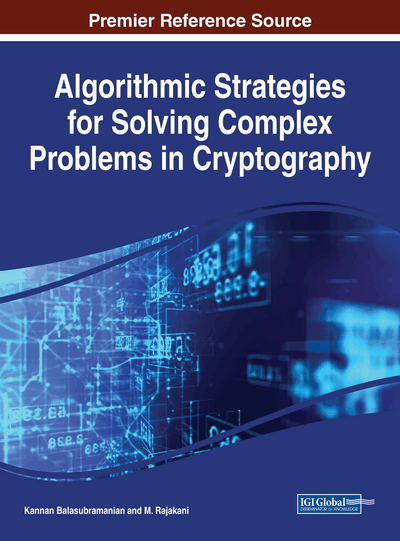# Problems in Cryptography and Cryptanalysis

Kannan Balasubramanian (Mepco Schlenk Engineering College, India) and Rajakani M. (Mepco Schlenk Engineering College, India)
DOI: 10.4018/978-1-5225-2915-6.ch002
Available
\$37.50
No Current Special Offers

## Abstract

The integer factorization problem used in the RSA cryptosystem, the discrete logarithm problem used in Diffie-Hellman Key Exchange protocol and the Elliptic Curve Discrete Logarithm problem used in Elliptic Curve Cryptography are traditionally considered the difficult problems and used extensively in the design of cryptographic algorithms. We provide a number of other computationally difficult problems in the areas of Cryptography and Cryptanalysis. A class of problems called the Search problems, Group membership problems, and the Discrete Optimization problems are examples of such problems. A number of computationally difficult problems in Cryptanalysis have also been identified including the Cryptanalysis of Block ciphers, Pseudo-Random Number Generators and Hash functions.
Chapter Preview
Top

## Problems In Cryptography

In search for more efficient and/or secure alternatives to established cryptographic protocols (such as RSA which is based on the factorization problem), there have been proposals for public key establishment protocols as well as with public key cryptosystems based on hard search problems from combinatorial (semi) group theory. These problems include the conjugacy search problem (Anshel et al.,1999; Ko, 2000), the homomorphism search problem (Grigoriev et al., 2006; Shpilrain, 2006a), the decomposition search problem (Cha et.al, 2001; Ko, 2000; Shpilrain, 2005) and the subgroup membership search problem (Shpilrain, 2006b). All these are problems of the following nature: given a property P and the information that there are objects with the property P, find at least one particular object with the property P from a pool S of objects.

The following problems have been considered difficult problems and used in cryptography.

The RSA Problem: Given a positive integer n that is a product of two distinct odd primes p and q and a positive integer e such that gcd(e,(p-1)(q-1)) =1 and an integer c, find an integer m such that mec(mod n).

The RSA Problem is that of finding the eth roots modulo a composite integer n. The underlying one-way function f(x) = xe (mod n) (f: ZnZn) is called the RSA function. Zn is the set of integers modulo n i.e., Zn = {0, 1, 2, …n-1}. Addition, Subtraction and Multiplication are performed modulo n. The inverse is f(x)-1 = xd (mod n), where de-1 (mod)

## Complete Chapter List

Search this Book:
Reset